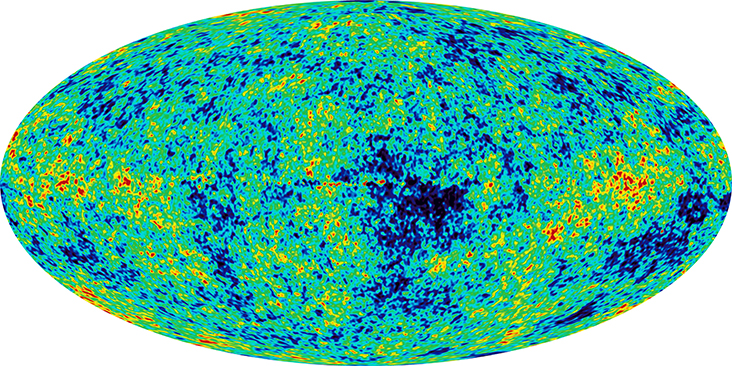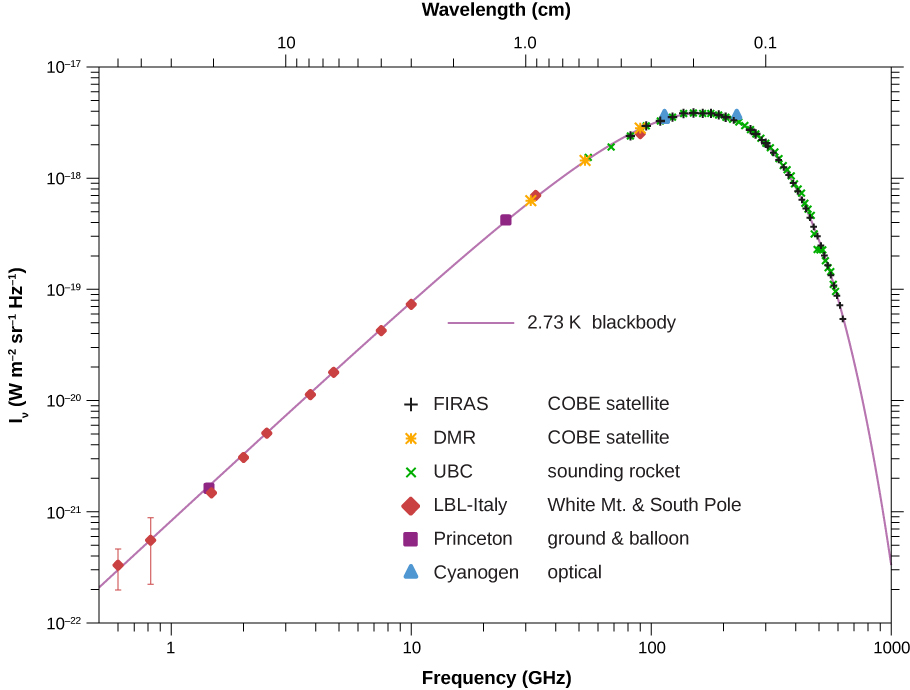# 11.7 Evolution of the early universe  (Page 3/10)

 Page 3 / 10

The predicted abundances of the elements in the universe provide a stringent test of the Big Bang and the Big Bang nucleosynthesis. Recent experimental estimates of the matter density from the Wilkinson Microwave Anisotropy Probe (WMAP) agree with model predictions. This agreement provides convincing evidence of the Big Bang model.

According to cosmological models, the Big Bang event should have left behind thermal radiation called the cosmic microwave background radiation (CMBR). The intensity of this radiation should follow the blackbody radiation curve ( Photons and Matter Waves ). Wien’s law states that the wavelength of the radiation at peak intensity is

${\lambda }_{\text{max}}=\frac{2.898\phantom{\rule{0.2em}{0ex}}×\phantom{\rule{0.2em}{0ex}}1{0}^{\text{−}3}\phantom{\rule{0.2em}{0ex}}\text{m-K}}{T},$

where T is temperature in kelvins. Scientists expected the expansion of the universe to “stretch the light,” and the temperature to be very low, so cosmic background radiation should be long-wavelength and low energy.

In the 1960s, Arno Penzias and Robert Wilson of Bell Laboratories noticed that no matter what they did, they could not get rid of a faint background noise in their satellite communication system. The noise was due to radiation with wavelengths in the centimeter range (the microwave region). Later, this noise was associated with the cosmic background radiation. An intensity map of the cosmic background radiation appears in [link] . The thermal spectrum is modeled well by a blackbody curve that corresponds to a temperature $T=2.7\text{K}$ ( [link] ).This map of the sky uses color to show fluctuations, or wrinkles, in the cosmic microwave background observed with the WMAP spacecraft. The Milky Way has been removed for clarity. Red represents higher temperature and higher density, whereas blue indicates lower temperature and density. This map does not contradict the earlier claim of smoothness because the largest fluctuations are only one part in one million.Intensity distribution of cosmic microwave background radiation. The model predictions (the line) agree extremely well with the experimental results (the dots). Frequency and brightness values are shown on a log axis. (credit: George Smoot/NASA COBE Project)

The formation of atoms in the early universe makes these atoms less likely to interact with light. Therefore, photons that belong to the cosmic background radiation must have separated from matter at a temperature T associated with 1 eV (the approximate ionization energy of an atom) . The temperature of the universe at this point was

${\text{k}}_{\text{B}}\text{T}\sim \text{1 eV}⇒T=\frac{\text{1 eV}}{8.617\phantom{\rule{0.2em}{0ex}}×\phantom{\rule{0.2em}{0ex}}1{0}^{5}\phantom{\rule{0.2em}{0ex}}\text{eV}\text{/}\text{K}}\sim {10}^{4}\phantom{\rule{0.2em}{0ex}}\text{K}.$

According to cosmological models, the time when photons last scattered oﬀ charged particles was approximately 380,000 years after the Big Bang. Before that time, matter in the universe was in the plasma form and the photons were “thermalized.”

## Antimatter and matter

We know from direct observation that antimatter is rare. Earth and the solar system are nearly pure matter, and most of the universe also seems dominated by matter. This is proven by the lack of annihilation radiation coming to us from space, particularly the relative absence of 0.511-MeV $\gamma$ rays created by the mutual annihilation of electrons and positrons. (Antimatter in nature is created in particle collisions and in ${\beta }^{+}$ decays, but only in small amounts that quickly annihilate, leaving almost pure matter surviving.)

fundamental note of a vibrating string
what are matter waves? Give some examples
according to de Broglie any matter particles by attaining the higher velocity as compared to light'ill show the wave nature and equation of wave will applicable on it but in practical life people see it is impossible however it is practicaly true and possible while looking at the earth matter at far
Manikant
Mathematical expression of principle of relativity
given that the velocity v of wave depends on the tension f in the spring, it's length 'I' and it's mass 'm'. derive using dimension the equation of the wave
What is the importance of de-broglie's wavelength?
he related wave to matter
Zahid
at subatomic level wave and matter are associated. this refering to mass energy equivalence
Zahid
it is key of quantum
Manikant
how those weight effect a stable motion at equilibrium
how do I differentiate this equation- A sinwt with respect to t
just use the chain rule : let u =wt , the dy/dt = dy/du × du/dt : wA × cos(wt)
Jerry
I see my message got garbled , anyway use the chain rule with u= wt , etc...
Jerry
de broglie wave equation
vy beautiful equation
chandrasekhar
what is electro statics
when you consider systems consisting of fixed charges
Sherly
Diagram of the derive rotational analog equation of v= u+at
what is carat
a unit of weight for precious stones and pearls, now equivalent to 200 milligrams.
LoNE
a science that deals with the composition, structure, and properties of substances and with the transformations that they undergo.
LoNE
what is chemistry
what chemistry ?
Abakar
where are the mcq
ok
Giorgi
acids and bases
Navya
How does unpolarized light have electric vector randomly oriented in all directions.
unpolarized light refers to a wave collection which has an equal distribution of electric field orientations for all directions
pro
In a grating, the angle of diffraction for second order maximum is 30°.When light of wavelength 5*10^-10cm is used. Calculate the number of lines per cm of the grating.
OK I can solve that for you using Bragg's equation 2dsin0over lander
ossyBy Madison ChristianBy OpenStaxBy OpenStaxBy Hoy WenBy Madison ChristianBy Jonathan LongBy OpenStaxBy Robert MurphyBy OpenStaxBy Subramanian Divya